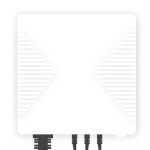# OWON PC321

Model PC321
Vendor OWON
Description 3-Phase clamp power meter
Exposes energy, voltage_l1, voltage_l2, voltage_l3, current_l1, current_l2, current_l3, energy_l1, energy_l2, energy_l3, reactive_energy_l1, reactive_energy_l2, reactive_energy_l3, power_l1, power_l2, power_l3, reactive_power_l1, reactive_power_l2, reactive_power_l3, linkquality
Picture## Options

How to use device type specific configuration

• `energy_precision`: Number of digits after decimal point for energy, takes into effect on next report of device. The value must be a number with a minimum value of `0` and with a with a maximum value of `3`

• `energy_calibration`: Calibrates the energy value (percentual offset), takes into effect on next report of device. The value must be a number.

## Exposes

### Energy (numeric)

Sum of consumed energy. Value can be found in the published state on the `energy` property. It’s not possible to read (`/get`) or write (`/set`) this value. The unit of this value is `kWh`.

### Voltage_l1 (numeric)

Phase 1 voltage. Value can be found in the published state on the `voltage_l1` property. It’s not possible to read (`/get`) or write (`/set`) this value. The unit of this value is `V`.

### Voltage_l2 (numeric)

Phase 2 voltage. Value can be found in the published state on the `voltage_l2` property. It’s not possible to read (`/get`) or write (`/set`) this value. The unit of this value is `V`.

### Voltage_l3 (numeric)

Phase 3 voltage. Value can be found in the published state on the `voltage_l3` property. It’s not possible to read (`/get`) or write (`/set`) this value. The unit of this value is `V`.

### Current_l1 (numeric)

Phase 1 current. Value can be found in the published state on the `current_l1` property. It’s not possible to read (`/get`) or write (`/set`) this value. The unit of this value is `A`.

### Current_l2 (numeric)

Phase 2 current. Value can be found in the published state on the `current_l2` property. It’s not possible to read (`/get`) or write (`/set`) this value. The unit of this value is `A`.

### Current_l3 (numeric)

Phase 3 current. Value can be found in the published state on the `current_l3` property. It’s not possible to read (`/get`) or write (`/set`) this value. The unit of this value is `A`.

### Energy_l1 (numeric)

Phase 1 energy. Value can be found in the published state on the `energy_l1` property. It’s not possible to read (`/get`) or write (`/set`) this value. The unit of this value is `kWh`.

### Energy_l2 (numeric)

Phase 2 energy. Value can be found in the published state on the `energy_l2` property. It’s not possible to read (`/get`) or write (`/set`) this value. The unit of this value is `kWh`.

### Energy_l3 (numeric)

Phase 3 energy. Value can be found in the published state on the `energy_l3` property. It’s not possible to read (`/get`) or write (`/set`) this value. The unit of this value is `kWh`.

### Reactive_energy_l1 (numeric)

Phase 1 reactive energy. Value can be found in the published state on the `reactive_energy_l1` property. It’s not possible to read (`/get`) or write (`/set`) this value. The unit of this value is `kVArh`.

### Reactive_energy_l2 (numeric)

Phase 2 reactive energy. Value can be found in the published state on the `reactive_energy_l2` property. It’s not possible to read (`/get`) or write (`/set`) this value. The unit of this value is `kVArh`.

### Reactive_energy_l3 (numeric)

Phase 3 reactive energy. Value can be found in the published state on the `reactive_energy_l3` property. It’s not possible to read (`/get`) or write (`/set`) this value. The unit of this value is `kVArh`.

### Power_l1 (numeric)

Phase 1 power. Value can be found in the published state on the `power_l1` property. It’s not possible to read (`/get`) or write (`/set`) this value. The unit of this value is `W`.

### Power_l2 (numeric)

Phase 2 power. Value can be found in the published state on the `power_l2` property. It’s not possible to read (`/get`) or write (`/set`) this value. The unit of this value is `W`.

### Power_l3 (numeric)

Phase 3 power. Value can be found in the published state on the `power_l3` property. It’s not possible to read (`/get`) or write (`/set`) this value. The unit of this value is `W`.

### Reactive_power_l1 (numeric)

Phase 1 reactive power. Value can be found in the published state on the `reactive_power_l1` property. It’s not possible to read (`/get`) or write (`/set`) this value. The unit of this value is `VAr`.

### Reactive_power_l2 (numeric)

Phase 2 reactive power. Value can be found in the published state on the `reactive_power_l2` property. It’s not possible to read (`/get`) or write (`/set`) this value. The unit of this value is `VAr`.

### Reactive_power_l3 (numeric)

Phase 3 reactive power. Value can be found in the published state on the `reactive_power_l3` property. It’s not possible to read (`/get`) or write (`/set`) this value. The unit of this value is `VAr`.

Link quality (signal strength). Value can be found in the published state on the `linkquality` property. It’s not possible to read (`/get`) or write (`/set`) this value. The minimal value is `0` and the maximum value is `255`. The unit of this value is `lqi`.